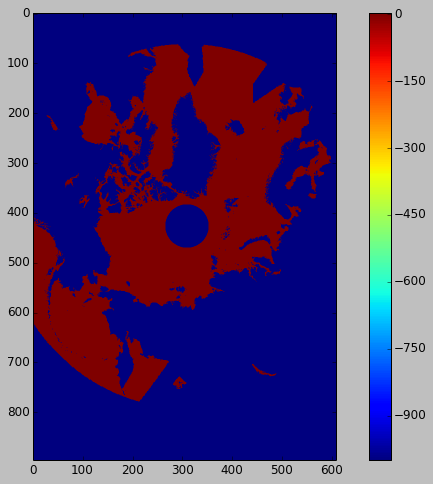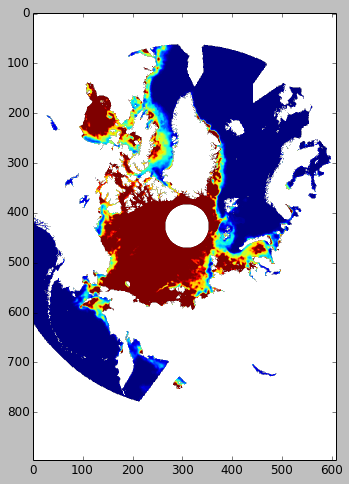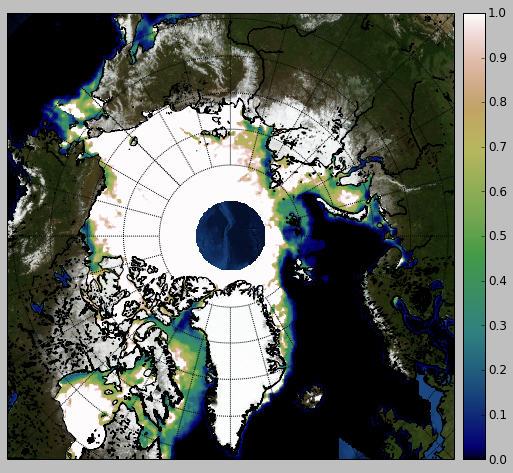# SMOS sea ice thickness

Acces SMOS Sea Ice Thickness data



Solution:

pydap

Sea ice thickens is one of the most important environmental variables in the Arctic, but unfortunately is one of the hardest to measure. Unlike sea ice concentration, which is measured by satellites operationally now for more than three decades, only recently we begin to obtain limited satellite sea ice thickness information from missions like ICESat, Cryosat-2 and SMOS. The later is not specifically dedicated to cryospheric applications, but turns out that its information can be used to obtain data about the thin sea ice.

In the following we learn how to access a SMOS dataset with the OpenNDAP protocol. This allows the analysis of some parts of the data while the complete dataset can stay on the remote server. Data hosted by ICDC.

We would need Python OpenNDAP client pydap.

In :
import pydap.client


Opening the dataset is straight forward:

In :
dataset=pydap.client.open_url('http://icdc.zmaw.de/thredds/dodsC/smos_icethickness_2014')


Let's have a look at the contents of the dataset

In :
dataset.keys() # Lets have a look at the contents

Out:
['y',
'x',
'longitude',
'latitude',
'time',
'sea_ice_thickness',
'saturation_ratio',
'nPair',
'land',
'ice_thickness_uncertainty',
'Tsurf',
'TB_uncertainty',
'TB',
'RFI_ratio']

You can access variables inside dataset by usual dictionary syntax:

In :
dataset['time'][:]

Out:
array([ 35076.,  35100.,  35124.,  35148.,  35172.,  35196.,  35220.,
35244.,  35268.,  35292.,  35316.,  35340.,  35364.,  35388.,
35412.,  35436.,  35460.,  35484.,  35508.,  35532.,  35556.,
35580.,  35604.,  35628.,  35652.,  35676.,  35700.,  35724.,
35748.,  35772.,  35796.,  35820.,  35844.,  35868.,  35892.,
35916.,  35940.,  35964.,  35988.,  36012.,  36036.,  36060.,
36084.,  36108.,  36132.,  36156.,  36180.,  36204.,  36228.,
36252.,  36276.,  36300.,  36324.,  36348.,  36372.,  36396.,
36420.,  36444.,  36468.,  36492.,  36516.,  36540.])

or as attributes:

In :
dataset.time[:]

Out:
array([ 35076.,  35100.,  35124.,  35148.,  35172.,  35196.,  35220.,
35244.,  35268.,  35292.,  35316.,  35340.,  35364.,  35388.,
35412.,  35436.,  35460.,  35484.,  35508.,  35532.,  35556.,
35580.,  35604.,  35628.,  35652.,  35676.,  35700.,  35724.,
35748.,  35772.,  35796.,  35820.,  35844.,  35868.,  35892.,
35916.,  35940.,  35964.,  35988.,  36012.,  36036.,  36060.,
36084.,  36108.,  36132.,  36156.,  36180.,  36204.,  36228.,
36252.,  36276.,  36300.,  36324.,  36348.,  36372.,  36396.,
36420.,  36444.,  36468.,  36492.,  36516.,  36540.])

Doesn't really look like a human readable time. Let's have a look at its units:

In :
dataset.time.attributes

Out:
{'standard_name': 'time', 'units': 'hours since 2010-01-01 00:00:00'}

Here we define function to convert from 'hours since' to something more understandable:

In :
import time
def ymd(y,m,d,offset_day):
"""Returns year,month,day string for an offset day"""
time_ymd=time.mktime((y, m, d, 1, 0, 0, 0, 0, 0))
ltime=time.localtime(time_ymd+offset_day*60*60*24)
return [str(ltime),str( '%(#)02d' % {'#':(int(ltime))}),str( '%(#)02d' % {'#':(int(ltime))})]


Get the latest date of the dataset and convert it (just to understand which day we are going to plot).

In :
ymd(2010,1,1,dataset.time[-1]/24) # Determine latest date

Out:
['2014', '03', '03']

Now we finlay get the lat/lon data, and retrieve the latest field of SMOS sea ice thickness:

In :
lats=dataset['latitude'].array[:,:]
lons=dataset['longitude'].array[:,:]
sit=array(dataset['sea_ice_thickness'][-1,:,:])

In :
imshow(sit[0,:,:])
colorbar()

Out:Data need a bit of work. We mask all -999 values and everything that is larger than 1 meter.

In :
sit[sit==-999.]=nan
sit[sit>1.]=1.0


In :
imshow(sitm[0,:,:])

Out:Use Basemap to make it much prettier :)

In :
from mpl_toolkits.basemap import Basemap

lat_ts=90.0
lat_0=90.0
lon_0=-45.0
sgn=1
width=7000000.
height=7000000.0
m = Basemap(width=width,height=height,resolution='h',\
projection='stere',lat_ts=lat_ts,lat_0=lat_0,lon_0=lon_0)
m.bluemarble()

x,y=m(lons,lats)

CS=m.pcolormesh(x,y,sitm[0,:,:],cmap=cm.gist_earth)#,ps,cmap=cm.jet,extend='max')
m.drawparallels(arange(-80.,81.,5.))#,labels=[1,0,0,0])
m.drawmeridians(arange(-180.,181.,15.))#,labels=[0,0,1,1])
m.drawcoastlines()
m.colorbar()
show()